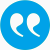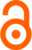#Strasser, Helmut. 2012. The covariance structure of conditional maximum likelihood estimates. Statistics & Risk Modelling 21 (4): 315-342.Show publication in ePubWU

BibTeX

## Abstract

In this paper we consider conditional maximum likelihood (cml) estimates for item parameters in the Rasch model under random subject parameters. We give a simple approximation for the asymptotic covariance matrix of the cml-estimates. The approximation is stated as a limit theorem when the number of item parameters goes to infinity. The results contain precise mathematical information on the order of approximation. The results enable the analysis of the covariance structure of cml-estimates when the number of items is large. Let us give a rough picture. The covariance matrix has a dominating main diagonal containing the asymptotic variances of the estimators. These variances are almost equal to the efficient variances under ml-estimation when the distribution of the subject parameter is known. Apart from very small numbers n of item parameters the variances are almost not affected by the number n. The covariances are more or less negligible when the number of item parameters is large. Although this picture intuitively is not surprising it has to be established in precise mathematical terms. This has been done in the present paper. The paper is based on previous results  of the author concerning conditional distributions of non-identical replications of Bernoulli trials. The mathematical background are Edgeworth expansions for the central limit theorem. These previous results are the basis of approximations for the Fisher information matrices of cml-estimates. The main results of the present paper are concerned with the approximation of the covariance matrices. Numerical illustrations of the results and numerical experiments based on the results are presented in Strasser . Read More: http://www.oldenbourg-link.com/doi/abs/10.1524/strm.2012.1125

## Tags

Press 'enter' for creating the tag

## Publication's profile

Status of publication Published WU Journal article Statistics and Risk Modeling FIN-A, VW-C English The covariance structure of conditional maximum likelihood estimates 21 4 2012 315 342 Y http://www.oldenbourg-link.com/doi/pdf/10.1524/strm.2012.1125?ai=2b1&ui=t4i&af=H

## Associations

People
Strasser, Helmut (Details)
Organization
Institute for Statistics and Mathematics IN (Details)